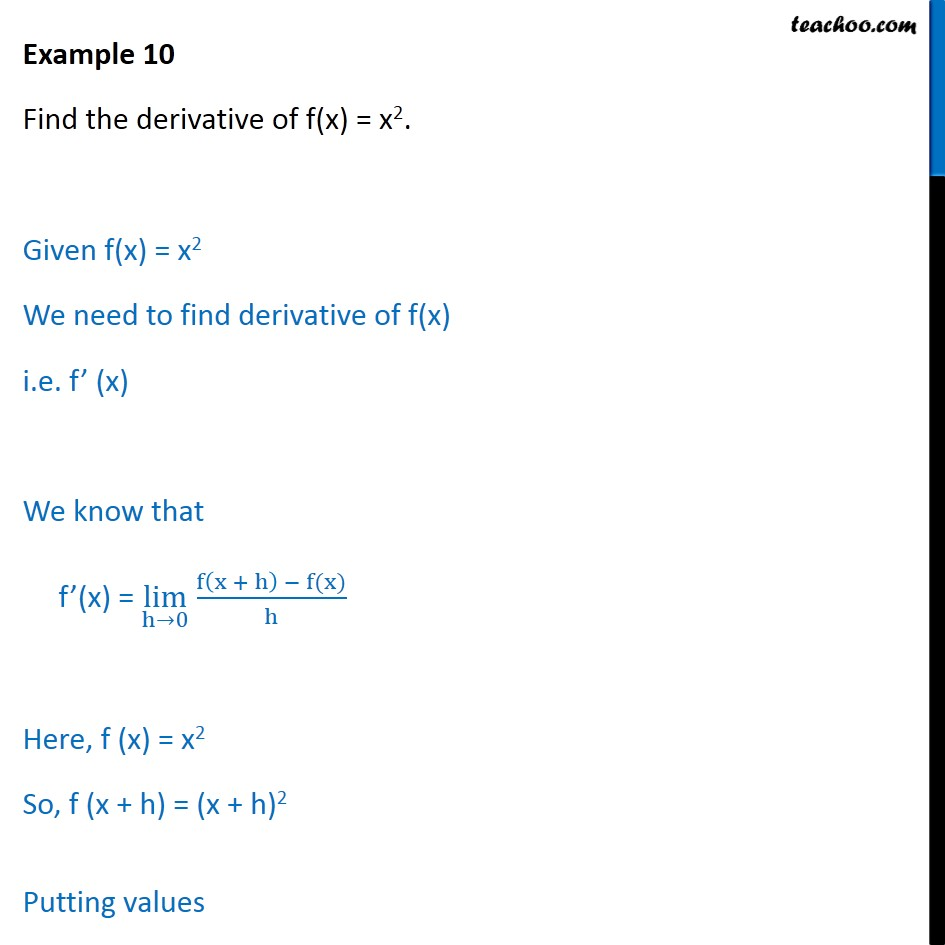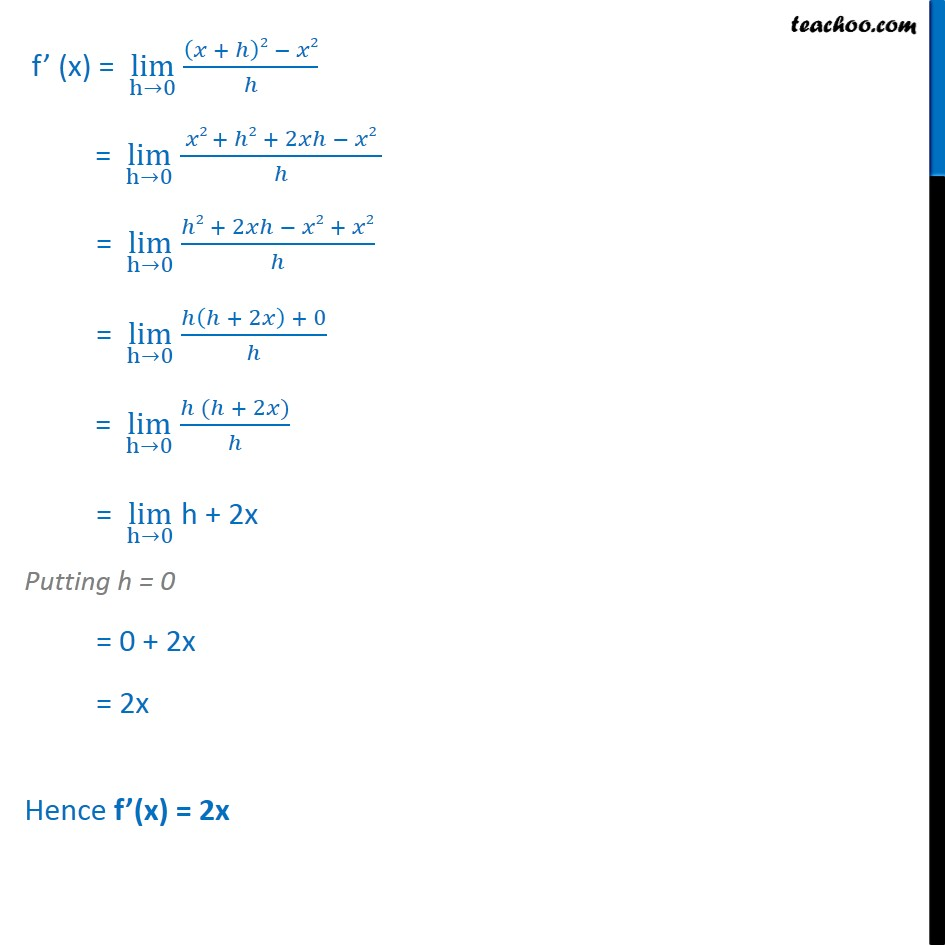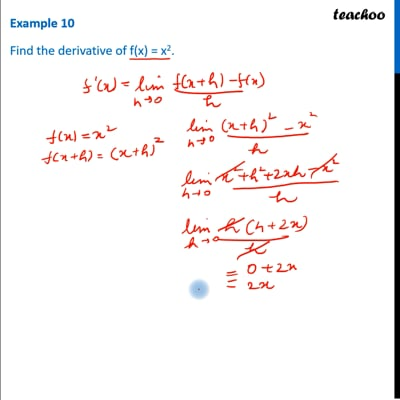Examples

Chapter 13 Class 11 Limits and Derivatives
Serial order wiseThis video is only available for Teachoo black users

Get live Maths 1-on-1 Classs - Class 6 to 12

### Transcript

Example 10 Find the derivative of f(x) = x2. Given f(x) = x2 We need to find derivative of f(x) i.e. f’ (x) We know that f’(x) = lim﷮h→0﷯ f﷮ x + h﷯ − f(x)﷯﷮h﷯ Here, f (x) = x2 So, f (x + h) = (x + h)2 Putting values f’ (x) = lim﷮h→0﷯ 𝑥 + ℎ﷯2 − 𝑥2﷮ℎ﷯ = lim﷮h→0﷯ 𝑥2 + ℎ2 + 2𝑥ℎ − 𝑥2 ﷮ℎ﷯ = lim﷮h→0﷯ ℎ2 + 2𝑥ℎ − 𝑥2 + 𝑥2﷮ℎ﷯ = lim﷮h→0﷯ ℎ ℎ + 2𝑥﷯ + 0﷮ℎ﷯ = lim﷮h→0﷯ ℎ (ℎ + 2𝑥)﷮ℎ﷯ = lim﷮h→0﷯ h + 2x Putting h = 0 = 0 + 2x = 2x Hence f’(x) = 2x